# Factoring Intro

//Factoring Intro
Factoring Intro 2017-11-13T22:01:52+00:00

The polynomial 2x + 8 can be modeled by the rectangle below.

Step 1 Gather the appropriate tiles.

Step 2 Arrange the tiles in the shape of a rectangle.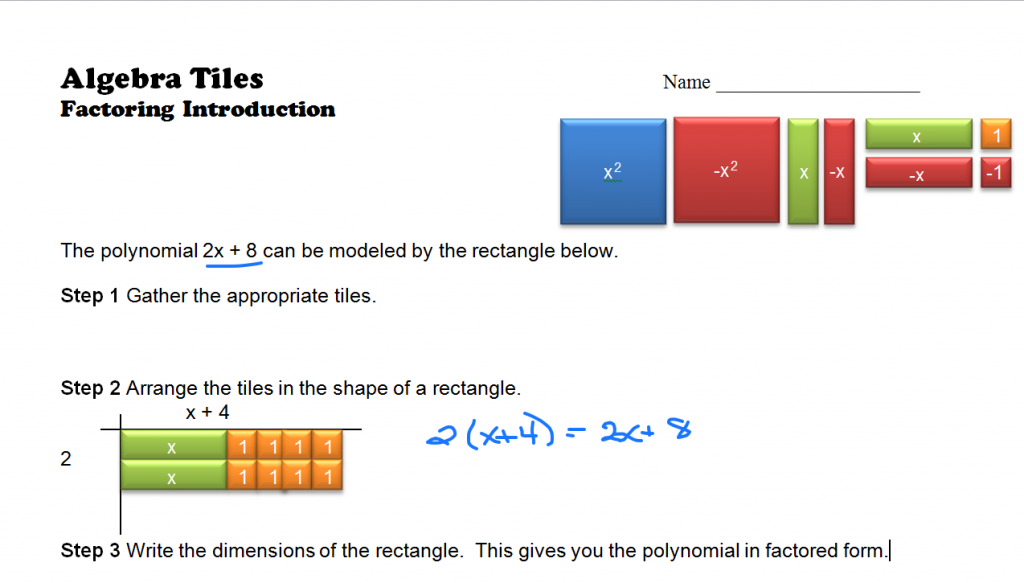Thus, 2x2 + 8 can be factored into 2(x + 4) . To check, simply use the distributive property to see if you get the original polynomial back.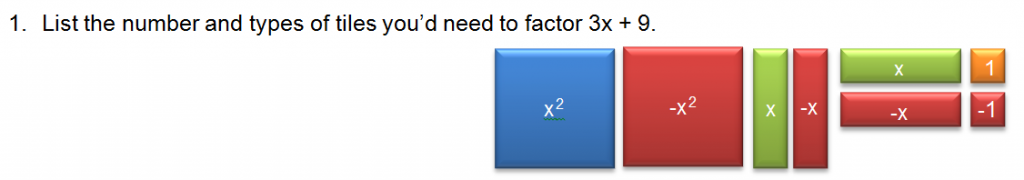Gather 3 of the positive x tiles and 9 of the positive one tiles. Then arrange them into a rectangle and write the dimensions of your rectangle.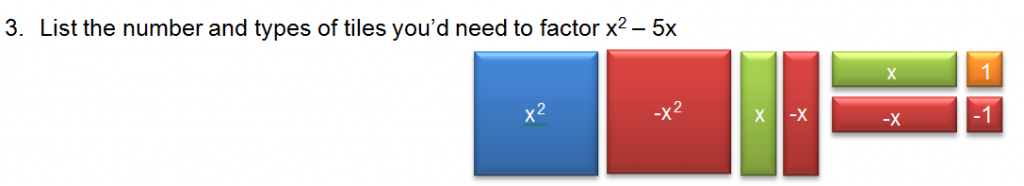Gather an x² tile and 5 negative x tiles. Arrange them into a rectangle and write the dimensions.

The above examples involved factoring binomials – polynomials with two terms. the examples below include trinomials.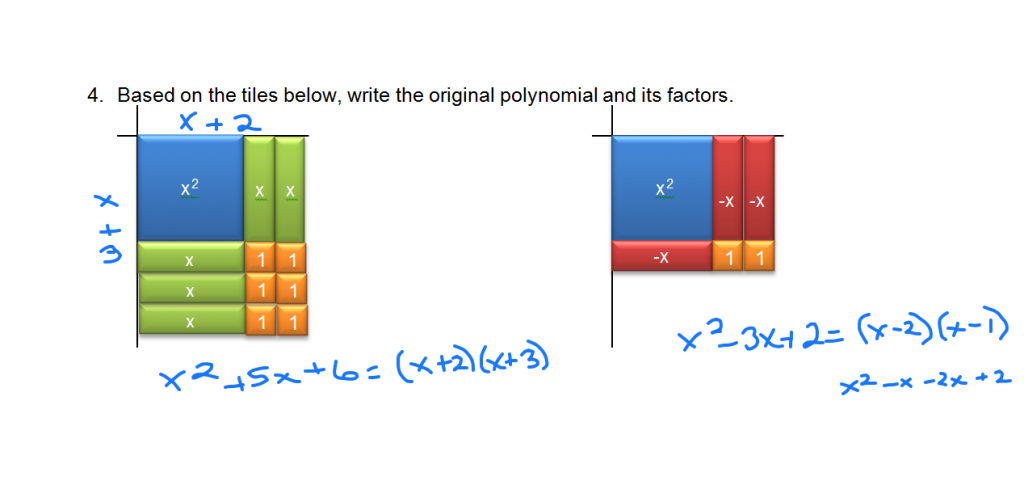Factor x² + 5x + 4 using Algebra Tiles.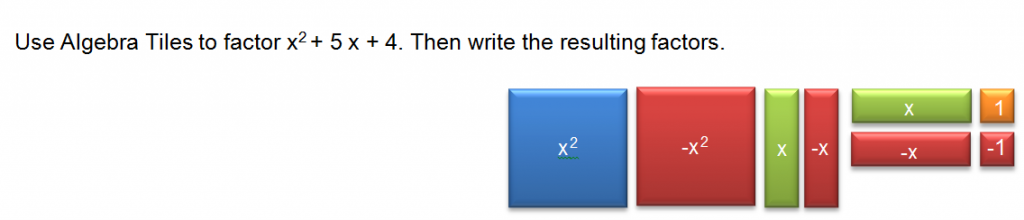Factor x² – 2x – 8.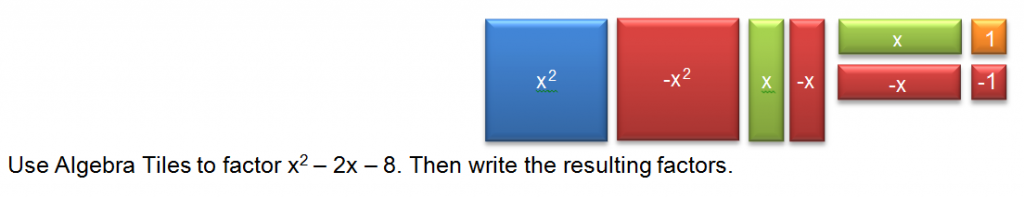A teacher demonstration file is available. Factoring using Algebra Tiles Introduction

A student activity is also included. Algebra Tiles factoring trinomials ws

Factoring Strategies